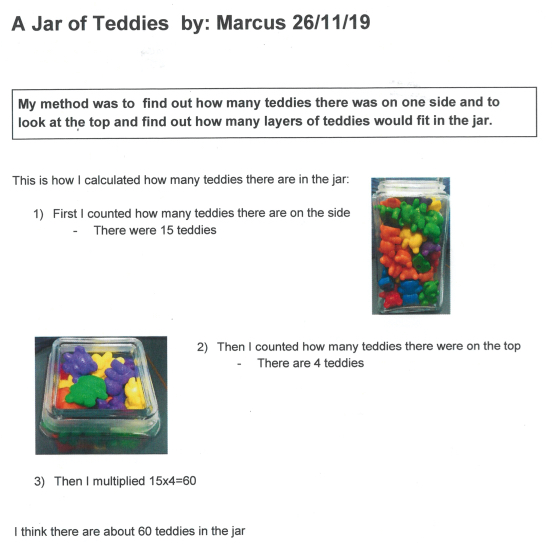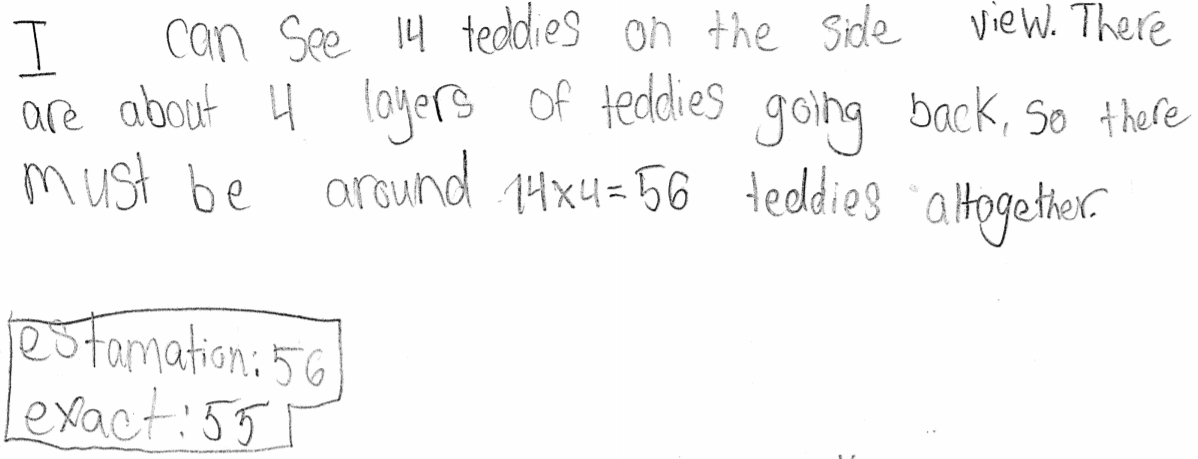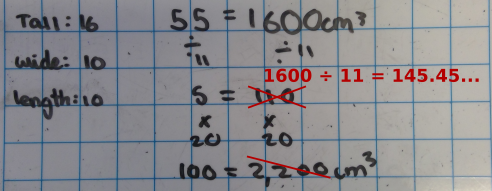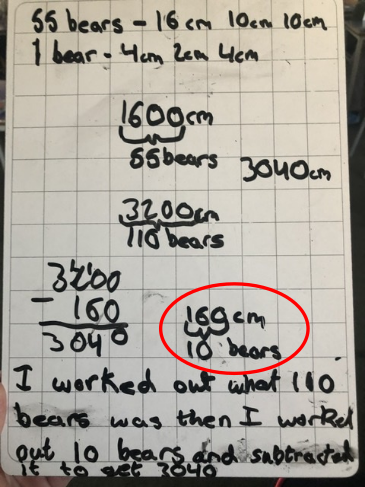#### You may also like### What's My Weight?

There are four equal weights on one side of the scale and an apple on the other side. What can you say that is true about the apple and the weights from the picture?### Whole Numbers Only

Can you work out how many of each kind of pencil this student bought?### Squaring the Circle

Bluey-green, white and transparent squares with a few odd bits of shapes around the perimeter. But, how many squares are there of each type in the complete circle? Study the picture and make an estimate.

# A Jar of Teddies

##### Age 7 to 14 Challenge Level:

Thank you for all of the solutions we received to this problem.

Click to see solutions which estimate the number of teddies in the jar

We had these solutions come in from the International School of Brussels:Jonathan:Click to see solutions for finding the size of the jar which would hold 100 teddies, using the volume of one teddy

Elmhurst School for Boys UK
The boys calculated a volume of 100 bears which was 3200cm cubed.
Then, they found the dimensions of different jars: 32 x 10 x 10 or 16 x 20 x 10 or 8 x 20 x 20 which would have sufficient capacity to hold all of the bears.

St. George’s
The jar needs to have a volume of approximately 3,200cm cubed. Height 32cm width 10cm length 10cm.

Click to see solutions for finding the size of the jar which would hold 100 teddies, using the the first jar and proportional reasoning

Miss Barlow's Class, Queen Anne's School, UK (error highlighted, but good method)Theo from Gospel Oak (UK) (error highlighted, but good method)160 cm$^3$ is actually only the space for 55$\div$10 = 5.5 teddies

Elmhurst School for Boys UK

The boys found the volume for 55 teddies which was 1600. They divided by 55 and multiplied by 100. This gave them 2900cm cubed approximately. Then they found jars which would create this capacity.

Click to see solutions for finding the number of teddies which would fit into a classroom, using the volume of one teddy

Churchfields Junior School (London)
Big Teddy = 16 x 19 x 12 = 3648cm$^3$.
Classroom = 280 x 710 x 840 = 166,992,000$^3$.
Using rounding we know the Teddy is approximately 3600cm$^3$ and the classroom is 166,000,000$^3$.
Therefore the volume of the classroom divided by the volume of bears gives us an answer of approximately 46,111 bears would fit in.
However, pupils did say this is assuming the classroom is completely empty. Moreover, we have cupboard space, which is not included in the volume and window sills that would affect the total amount.

Elmhurst School for Boys UK
First we found the volume of a teddy bear which is 3648cm cubed. The length of our room was 7m, the width was 3m and the height was 2.8m. We calculated the volume for this which was 58.8 cubic metres. We divided 58.8 million cubic centimetres by 3648 and we got 16118 with a remainder which we rounded to 16119.

How many teddies can fit in our classroom?
First we found the volume of the classroom. Volume of Classroom: 2.3 x 8.5 .8 = 1564 m$^3$.
Then we realised we had to convert this into cm because the measurements of the teddy were in cm. Volume of classroom = 156, 400,000 cm$^3$.
Then we calculated the volume of the teddy. Volume of teddy: 19 x 12 x 16 = 3648 cm$^3$ .
Then we calculated the number of teddies by: Volume of classroom divided by Volume of teddy.
156,400,000/3648 = 42,873 teddy bears to the nearest whole. We rounded our answer.
We decided not to include the sloped part of the roof and the cupboards. It was a lot of teddy bears. We could have fitted more in if we had squashed them together so it would have been more accurate if we had been using a cube.

St. George’s
We roughly measured our class and estimated the volume, Class is 800cm by 650cm by 300cm.
We worked out the volume of the teddy. Then, we rounded to help us divide. We think we can fit 39,000 teddies in.

We estimate it would take 41,000 teddies to fill our classroom which has a volume of 150m cubed. So we rounded the measurements of teddy to calculate the volume of teddy.
We then calculated volume of classroom and then converted in cm. Then divided volume of class by volume of teddy.

Some people raised concerns about using the volume of one teddy:
Haze, Iromic Schule, Germany:

But then you have to account for the teddie-air ratio
Sophie, Woodland School Basildon, UK:
The bears will compress so the volume will be variable
What about using the first jar of teddies and proportional reasoning to estimate the number of teddies that would fit in your classroom?

Click to see questions which could be investigated

St George's
How many Bears would fit in the classrooms in KS2? We could share the answers from today and add them together!
How long would it take to physically fill the rooms with teddies?
If a square classroom had a width of 17m and 3m tall how many bears would fit?

Meg, Gosel Oak
How many small teddy bears can fit in a big teddy bear?

Newbottle and Charlton Primary School
How many of the small bears could fit in the classroom?
How many big bears could in a mini bear?
How many dice could you fit in the classroom?

How many chocolate bars fill your office?
How many pencils can you fit in your bin?
How many pigs can fit in your school?
How many gluesticks can you fit in an average pencil case?

Class 6B, Scotby, England
How many 5ft tall children can you fit in a double decker bus?
How many standard size hb pencils can you fit in 1 single layer class tray?

Click to see solutions which investigate their own creative questions

Annabelle and Darcie and Lauren, Isabelle and Chloe, Jersey College for Girls
The question we wanted to answer was how many large teddies could we fit around the equator of the earth.  To do this we used our knowledge that the width of the teddy is 19cm and we googled the circumference of the earth
which is 40,075km.  We noticed to divide these two numbers we would have to convert 40,075km to cm so they have the same units.  So we multiplied it by 100,000 and we got the answer 4,007,500,000cm.  Then we divided that answer
by 19cm and got 210921052.6 teddies.  As you can't have part of a teddy bear and we couldn't fit another teddy bear in we rounded down so we can fit 210,921,052 teddy bears around the equator.

Click here to see Lauren, Isabelle and Chloe's diagrams

Scarlett and Mae, Jersey College for Girls